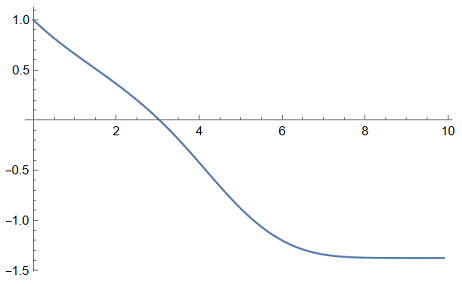# On solving a first order nonlinear differential equation

It all starts with this Cauchy problem:

$$\begin{cases} \sin(2x(t)) -\cos(3x'(t)) = x(t) + x'(t) \\ x(0) = 1 \\ \end{cases} \quad \quad \text{with} \; t \in [0,10]\,.$$

Not knowing which way to turn, I started Mathematica:

Clear[t, x];
xsol = NDSolveValue[{Sin[2 x[t]] - Cos[3 x'[t]] == x[t] + x'[t], x == 1}, x,
{t, 0, 10}, Method -> {"EquationSimplification" -> "Residual"}];
Plot[xsol[t], {t, 0, 10}, PlotRange -> All]So, seeing no hope of being able to apply Euler or Runge-Kutta-4, I thought of finite differences:

t = x = ConstantArray[0, 101];
{dt, t[], x[]} = {0.1, 0, 1};

Do[t[[k]] = t[[k - 1]] + dt;
res = Sin[2 y] - Cos[3 (y - x[[k - 1]])/dt] - y - (y - x[[k - 1]])/dt;
x[[k]] = NSolve[res == 0, y, Reals][[1, 1, 2]],
{k, 2, 101}];

ListLinePlot[Transpose[{t, x}], PlotRange -> All]which works great but… can you do better? Is it really necessary to use NSolve[]?

In environments without a similar solver how could this differential equation be solved?

@Lutz Lehmann: I think I have to eat some pasta before I get such good ideas!

Thanks a lot, I've already tried with Euler and everything works wonderfully.

You can force a solvable explicit differential equation by taking the derivative of the equation $$2\cos(2x)x'+3\sin(3x')x''=x'+x''\\~\\ x''=\frac{(2\cos(2x)-1)x'}{1-3\sin(3x')}$$ For the initial condition you need to once select a solution $$x'(0)$$ of the original non-linear implicit equation.